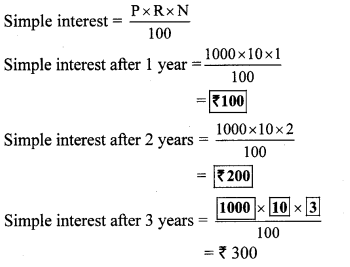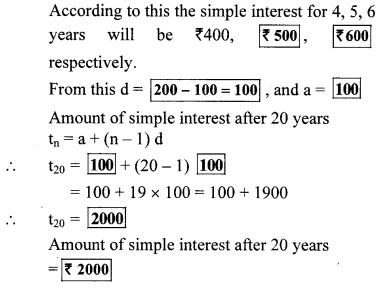# Maharashtra Board 10th Class Maths Part 1 Problem Set 3 Solutions Chapter 3 Arithmetic Progression

Balbharti Maharashtra State Board Class 10 Maths Solutions covers the Problem Set 3 Algebra 10th Class Maths Part 1 Answers Solutions Chapter 3 Arithmetic Progression.

## Problem Set 3 Algebra 10th Std Maths Part 1 Answers Chapter 3 Arithmetic Progression

Question 1.
Choose the correct alternative answer for each of the following sub questions.

i. The sequence – 10,- 6,- 2, 2, …
(A) is an A.P. Reason d = – 16
(B) is an A.P. Reason d = 4
(C) is an A.P. Reason d = – 4
(D) is not an A.P.
(B)

ii. First four terms of an A.P. are …, whose first term is -2 and common difference is -2.
(A) -2, 0, 2, 4
(B) -2, 4,- 8, 16
(C) -2, -4, -6, -8
(D) -2,-4, -8, -16
(C)

iii. What is the sum of the first 30 natural numbers?
(A) 464
(B) 465
(C) 462
(D) 461
(B)

iv. For an given A.P. t7 = 4, d = – 4, then a = ………
(A) 6
(B) 7
(C) 20
(D) 28
(D)

v. For an given A.P. a = 3.5, d = 0, n = 101, then tn = ….
(A) 0
(B) 3.5
(c) 103.5
(D) 104.5
(B)

vi. In an A.P. first two terms are – 3, 4, then 21st term is ….
(A) -143
(B) 143
(C) 137
(D) 17
(C)

vii. If for any A.P. d = 5, then t18 – t13 = ….
(A) 5
(B) 20
(C) 25
(D) 30
(C)

viii. Sum of first five multiples of 3 is …
(A) 45
(B) 55
(C) 15
(D) 75
(A)

ix. 15, 10, 5, … In this A.P. sum of first 10 terms is…
(A) -75
(B) -125
(C) 75
(D) 125
(A)

x. In an A.P. 1st term is 1 and the last term is 20. The sum of all terms is 399, then n = ….
(A) 42
(B) 38
(C) 21
(D) 19
(B)

Hints: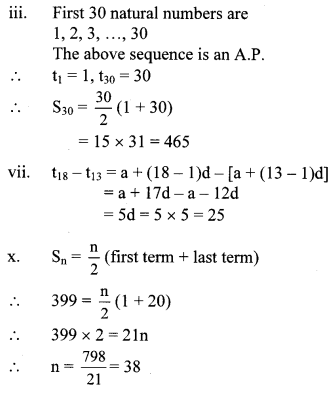Question 2.
Find the fourth term from the end in an
A.P.: -11, -8, -5, …, 49.
Solution:
The given A.P. is
-11,-8,-5, ……. 49
Reversing the A.P., we get 49, …, -5, -8, -11
Here, a = 49, d = -11 -(-8) = -11 + 8 = -3
Since, tn = a + (n – 1)d
∴ t4 = 49 + (4 – 1)(-3)
= 49 + (3) (-3)
= 49 – 9
= 40
∴ Fourth term from the end in the given A.P. is 40.
[Note: If an AY. is reversed, then the resulting sequence is also an A.P.]

Question 3.
In an A.P. the 10th term is 46, sum of the 5th and 7th term is 52. Find the A.P.
Solution:
For an A.P., let a be the first term and d be the common difference.
t10 = 46, t5 + t7 = 52 …[Given]
Since, tn = a + (n – 1)d
∴ t10 = a + (10 – 1)d
∴ 46 = a + 9d
i. e. a + 9d = 46 …(i)
Also, t5 + t7 = 52
∴ a + (5 – 1)d + a + (7 – 1)d = 52
∴ a + 4d + a + 6d = 52
∴ 2a + 10d = 52
∴ 2 (a + 5d) = 52
∴ a + 5d = $$\frac { 52 }{ 2 }$$
∴ a + 5d = 26 …(ii)
Subtracting equation (ii) from (i), we getSubstituting d = 5 in equation (ii), we get
a + 5(5) = 26
∴ a + 25 = 26
∴ a = 26 – 25 = 1
t1 = a = 1
t2 = t1 + d = 1 + 5 = 6
t3 = t2 + d = 6 + 5 = 11
t4 = t3 + d = 11 + 5 = 16
The required A.P. is 1,6,11,16,….

Question 4.
The A.P. in which 4th term is -15 and 9th term is -30. Find the sum of the first 10 numbers.
Solution:
t4 = -15, t9 = – 30 …[Given]
Since, tn = a + (n – 1)d
∴ t4 = a + (4 – 1)d
∴ – 15 = a + 3d
i. e. a + 3d = -15 …(i)
Also, t9 = a + (9 – 1)d
∴ -30 = a + 8d
i.e. a + 8d = -30 …(ii)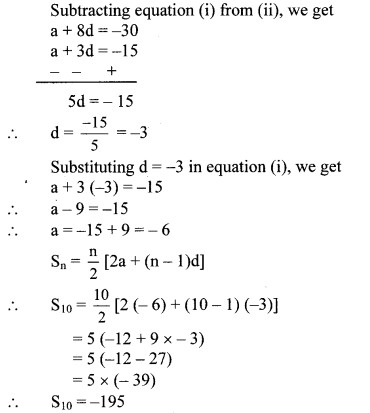∴ The sum of the first 10 numbers is -195.

Question 5.
Two given A.P.’s are 9, 7, 5, … and 24, 21, 18, … If nth term of both the .progressions are equal then find the value of n and n,h term.
Solution:
The first A.P. is 9, 7, 5,…
Here, a = 9, d = 7- 9 = -2
∴nth term = a + (n – 1)d
= 9 + (n – 1) (-2)
= 9 – 2n + 2
= 11 – 2n
The second A.P. is 24, 21, 18, …
Here, a = 24, d = 21 – 24 = – 3
∴ nth term = a + (n – 1)d
= 24 + (n – 1) (-3)
= 24 – 3n + 3
= 27 – 3n
Since, the nth terms of the two A.P.’s are equal.
∴ 11 – 2n = 27 – 3n
∴ 3n – 2n = 27 – 11
∴ n = 16
∴ t16 = 9 + (16 – 1) (-2)
= 9 + 15 × (-2)
= 9 – 30
∴ t16 = -21
∴ The values of n and nth term are 16 and -21 respectively.

Question 6.
If sum of 3rd and 8th terms of an A.P. is 7 and sum of 7th and 14th terms is -3, then find the 10th term.
Solution:
for an A.P., let a be the first term and d be the common difference.
According to the first condition,
t3 + tg = 7
∴ a + (3 – 1) d + a + (8 – 1)d = 7 …[∵ tn = a + (n – 1)d]
∴ a + 2d + a + 7d = 7
∴ 2a + 9d = 7 …(i)
According to the second condition,
t7 + t14 = -3
∴ a + (7 – 1)d + a + (14 – 1 )d = -3
∴ a + 6d + a + 13d = -3
∴ 2a + 19 d = – 3 …(ii)
Subtracting equation (i) from (ii), we get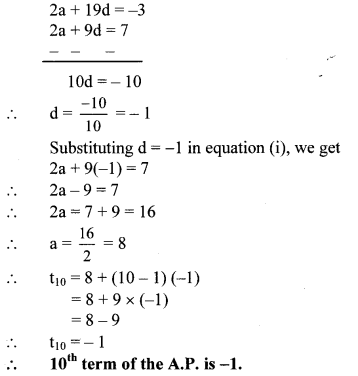Question 7.
In an A.P. the first term is -5 and last term is 45. If sum of all numbers in the A.P. is 120, then how many terms are there? What is the common difference?
Solution:
Let the number of terms in the A.P. be n and the common difference be d.
Then, a = -5, tn = 45, Sn = 120
Since, tn = a + (n – 1)d
∴ 45 = -5 + (n – 1)d
∴ 45 + 5 = (n – 1)d
∴ (n – 1)d = 50 …(i)Substituting n = 6 in equation (i), we get
(6 – 1)d = 50
∴ 5d = 50
∴ d = $$\frac { 50 }{ 5 }$$ = 10
∴ There are 6 terms in the A.P. and the common difference is 10.

Alternate Method:
Let the number of terms in the A.P. be n.
Then, t1 = a = -5, tn = 45, Sn = 120∴ There are 6 terms in the A.P. and the common difference is 10.

Question 8.
Sum of 1 to n natural numbers is 36, then find the value of n.
Solution:
The natural numbers from 1 to n are
1,2, 3, ……, n.
The above sequence is an A.P.
∴ a = 1, d = 2 – 1 = 1
Sn = 36 …[Given]
Now, Sn = $$\frac { n }{ 2 }$$ [2a + (n – 1)d]
∴ 36 = $$\frac { n }{ 2 }$$ [2(1) + (n – 1)(1)]
∴ 36 = $$\frac { n }{ 2 }$$ (2 + n – 1)
∴ 36 × 2 = n (n + 1)
∴ 72 = n (n + 1)
∴ 72 = n2 + n
∴ n2 + n – 72 = 0
∴ n2 + 9n – 8n – 72 = 0
∴ n(n + 9) – 8 (n + 9) = 0
∴ (n + 9) (n – 8) = 0
∴ n + 9 = 0 or n – 8 = 0
∴ n = -9 or n = 8
But, n cannot be negative.
∴ n = 8
∴ The value of n is 8.

Question 9.
Divide 207 in three parts, such that all parts are in A.P. and product of two smaller parts will be 4623.
Solution:
Let the three parts of 207 that are in A.P. be
a – d, a, a + d
According to the first condition,
(a – d) + a + (a + d) = 207
∴ 3a = 207
∴ a = $$\frac { 207 }{ 3 }$$
∴ a = 69 …(i)
According to the second condition,
(a – d) × a = 4623
∴ (69 – d) × 69 = 4623 …[From (i)]
∴ 69 – d = $$\frac { 4623 }{ 69 }$$
∴ d = 69 – 67
∴ d = 2
∴ a – d = 69 – 2 = 67
a = 69
a + d = 69 + 2 = 71
∴ The three parts of 207 that are in A.P. are 67, 69 and 71.

Question 10.
There are 37 terms in an A.P., the sum of three terms placed exactly at the middle is 225 and the sum of last three terms is 429. Write the A.P.
Solution:
Since, there are 37 terms in the A.P.Substituting d = 4 in equation (i), we get
3a + 54(4) = 225
∴ 3a + 216 = 225
∴ 3a = 225 – 216
∴ 3a = 9
∴ a = $$\frac { 9 }{ 3 }$$ = 3
∴The required A. P. is
a, a + d, a + 2d, a + 3d, …., a + (n – 1)d
i.e. 3, 3 + 4,3 + 2 × 4, 3 + 3 × 4,…, 3 + (37 – 1)4
i.e. 3, 7,11,15, …,147

Question 11.
If first term of an A.P. is a, second term is b and last term is c, then show that sum of all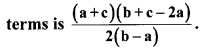Solution:Question 12.
If the sum of first p terms of an A.P. is equal to the sum of first q terms then show that the sum of its first (p + q) terms is zero, (p ≠ q)
Solution:
For an A.P., let a be the first term and d be the common difference.
The sum of first n terms of an A.P. is given by
Sn = [2a + (n – 1)d]
According to the given condition,
Sp = Sq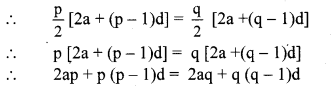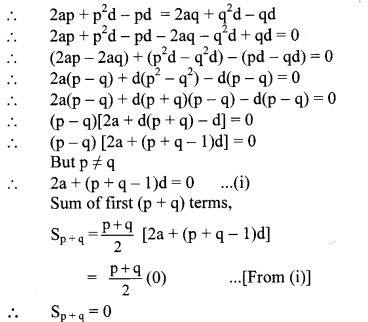∴ The sum of the first (p + q) terms is zero

Question 13.
If m times the mth term of an A.P. is equal to n times nth term, then show that the (m + n)th term of the A.P. is zero.
Solution:
According to the given condition,
mtm = ntn
∴ m[a + (m – 1)d] = n[a + (n – 1)d]
∴ ma + md(m – 1) = na + nd(n- 1)
∴ ma + m2d – md = na + n2d – nd
∴ ma + m2d – md – na – n2d + nd = 0
∴ (ma – na) + (m2d – n2d) – (md – nd) = 0
∴ a(m – n) + d(m2 – n2) – d(m – n) = 0
∴ a(m – n) + d(m + n) (m – n) – d(m – n) = 0
∴ (m – n)[a + (m + n – 1) d] = 0
∴ [a+ (m + n – 1)d] = 0 …[Dividing both sides by (m – n)]
∴ t(m+n) = 0
∴ The (m + n)th term of the A.P. is zero.

Question 14.
₹ 1000 is invested at 10 percent simple interest. Check at the end of every year if the total interest amount is in A.P. If this is an A.P. then find interest amount after 20 years. For this complete the following activity.
Solution: